Phase transition, elastic and electronic properties of topological insulator Sb2Te3 under pressure: First principle study
Institute of Atomic and Molecular Physics, College of Physical Science and Technology, Sichuan University, Chengdu 610065, China
Key Laboratory of High Energy Density Physics and Technology of Ministry of Education, College of Physical Science and Technology, Sichuan University, Chengdu 610064, China
National Key Laboratory of Shock Wave and Detonation Physics, Institute of Fluid Physics, Chinese Academy of Engineering Physics, Mianyang 621900, China

† Corresponding author. E-mail: ycheng66@qq.com

‡ Corresponding author. E-mail: xrchen66@qq.com

Project supported by the National Natural Science Foundation of China (Grant Nos. 11204192 and 11174214) and Jointly supported by the National Natural Science Foundation of China and the China Academy of Engineering Physics (NSAF) (Grant No. U1430117).

Abstract
Abstract

The phase transition, elastic and electronic properties of three phases (phase I, II, and III) of Sb2Te3 are investigated by using the generalized gradient approximation (GGA) with the PBESOL exchange–correlation functional in the framework of density-functional theory. Some basic physical parameters, such as lattice constants, bulk modulus, shear modulus, Young’s modulus, Poisson’s ratio, acoustic velocity, and Debye temperature Θ are calculated. The obtained lattice parameters under various pressures are consistent with experimental data. Phase transition pressures are 9.4 GPa (I → II) and 14.1 GPa (II → III), which are in agreement with the experimental results. According to calculated elastic constants, we also discuss the ductile or brittle characters and elastic anisotropies of three phases. Phases I and III are brittle, while phase II is ductile. Of the three phases, phase II has the most serious degree of elastic anisotropy and phase III has the slightest one. Finally, we investigate the partial densities of states (PDOSs) of three phases and find that the three phases possess some covalent features.

1. Introduction

Antimony telluride (Sb2Te3) is a member of the (Bi, Sb)2(Te, Se)3 family with a narrow band gap. These layered chalcogenides are typical thermoelectric materials. Hence these materials and their alloys are widely studied. Compared with bulk Bi2Te3 alloy, the thin-film thermoelectric material demonstrates a significant enhancement in the figure of merit at 300 K. First-principle calculations show that a uniaxial pressure applied perpendicularly to the plane of the layered structure substantially improves the power factor of Sb2Te3. Calculations also show that under a uniaxial pressure of 1.5 GPa, multiple valence band maximal value occurs which may enhance thermoelectric properties. Owing to the fast speed, excellent endurance, and low-programming energy, Sb2Te3 is a promising candidate for phase change random access memories (PRAMs). Recently, chalcogenides (Sb2Te3, Bi2Te3, and Bi2Se3) have received attention again, because they are predicted to be three-dimensional (3D) topological insulators. The topological insulator is a new class of material, which features single Dirac cone at the Γ point.

Pressure can reduce interatomic distances and tune material properties, so high pressure study is helpful to understand properties of materials. Studies of these layered chalcogenides have shown that each of these chalcogenides presents a pressure-induced superconductivity.[12,13] Under ambient conditions, Sb2Te3 has a rhombohedral structure (R3m, α-Sb2Te3, phase I). It is a long puzzle to determine the crystalline structures of Sb2Te3 at high pressures. Through the x-ray diffraction (XRD) experiments, Zhao et al. reported that at about 9.3 GPa, Sb2Te3 started to transform from the rhombohedral structure to a monoclinic C2/m, and the transition to the monoclinic C2/m structure was completed at 12.3 GPa. At about 15.1 GPa, another disordered monoclinic C2/m structure emerged and this disordered C2/m structure was completely formed at 18.9 GPa. Then, it transformed to a disordered body-centered cubic Im3m structure at about 19.8 GPa and this transformation was completed at above 29.7 GPa. Thus, Sb2Te3 underwent a sequence of phase transitions as follows: rhombohedral R3m structure → (9.3 GPa) monoclinic C2/m structure → (15.1 GPa) disordered monoclinic C2/m structure → (19.8 GPa) disordered body-centered cubic Im3m structure. In the following mentioned experiments, we just focus on the pressure under which the new phase emerges, but will not mention the pressure ranges of coexistence at all.

Using XRD experimental technique, Souza et al. observed two high pressure phases at pressures of 9.8 GPa and 15.2 GPa, respectively. They determined that the first high pressure phase was of a monoclinic C2/m structure and the second was of an ordered C2/c structure. Recently, Ma et al. also explored the high pressure behaviors of Sb2Te3 at pressures of up to 52.7 GPa through angle-dispersive synchrotron x-ray powder diffraction (XRPD) technique in a diamond anvil cell and their experimental results showed that the phase (R3m, α-Sb2Te3) transformed into phase II (monoclinic sevenfold C2/m, β-Sb2Te3) and phase III (monoclinic eightfold C2/c, γ-Sb2Te3) around 8.0 GPa and 13.2 GPa, respectively, and at above 21.6 GPa, a disordered body-centered cubic Im3m structure (phase IV, δ-Sb2Te3) emerged. This phase sequence is consistent with the transition sequence of Bi2Te3.

In our present work, using the plane-wave pseudopotential density functional theory (DFT) method as implemented in the Cambridge Serial Total Energy Package (CASTEP) code,[22,23] we investigate the phase transition and elastic properties of Sb2Te3 under pressure. Since the calculations of the disordered bcc phase are not possible in the CASTEP code, we just discuss the phases I, II, and III in our following calculations. The remaining parts of this paper are organized as follows. In Section 2, the computation details are presented. The results and discussion are shown in Section 3. Then electronic properties are discussed in Section 4. Finally, a summary of our results is given in Section 5.

2. Computation details

Equilibrium properties, such as bulk moduli, phonon frequencies and magnetisms are sensitive to lattice constants. Generally speaking, the lattice constants predicted from PBESOL[24,25] exchange–correlation functional were better than those predicted from the local spin density approximation (LSDA), the Perdew–Burke–Ernzerh (PBE) version of the generalized gradient approximation (GGA) or the Tao–Perdew–Staroverov–Scuseria (TPSS) meta-GGA. Thus the PBESOL was employed for describing the exchange–correlation energy of electrons. The ultrasoft “on the fly” pseudopotential was chosen for the interaction of the electrons with the ion cores, and pseudo atomic calculations were performed for Sb-5s25p3 and Te-5s25p4. The electronic wave functions were expanded in a plane wave basis set with an energy cut-off of 260 eV. For the Brillouin-zone sampling, we used the Monkhorst–Pack meshs 8×8×8, 8×8×3 and 3×3×2 for phases I, II, and III, respectively. In the geometry optimization, criteria of convergence were set to be 5×10−6 eV/atom for energy, 0.01 eV/Å for force, 5×10−4 Å for ionic displacement, and 0.02 GPa for stress. In calculations of elastic properties, criteria of convergence were set to be 1×10−6 eV/atom for energy, 0.002 eV/Å for the maximum force, 1×10−4 for the maximum ionic displacement, and 0.003 GPa for the maximum strain amplitude. These parameters are carefully tested and sufficient to lead to a well converged total energy.

3. Results and discussion
3.1. Structural properties

The experimental atomic positions for all phases are presented as follows. The atomic positions of phase I are Sb occupied 6c Wyckoff site (0,0,0.3988), Te1 located at 3a Wyckoff site (0,0,0), and Te2 located at 6c Wyckoff site (0,0,0.7872) under ambient conditions. At 13.2 GPa, the atomic positions of phase II are Sb1 with 4i Wyckoff site (0.1971,0,0.2079), Sb2 with 4i Wyckoff site (0.4599,0,0.2340), Te1 with 4i Wyckoff site (0.2321,0,0.3965), Te2 with 4i Wyckoff site (0.0451,0,0.6106), and Te3 with 4i Wyckoff site (0.3470,0,0.9853). The atomic positions of phase III are Sb with 8 f Wyckoff site (0.278,0.1429,0.8396), Te1 with 8f Wyckoff site (0.5668,0.3853,0.9363), and Te2 with 4e Wyckoff site (0.5,-0.0921,0.75) at 20.5 GPa. These structures are also shown in Fig. 1. All calculations in our paper are rhombohedral representation for phase I and primitive cells for phase II and III. The geometry optimizations are performed for phases I, II, and III under pressure. The relaxed lattice parameters of unit cell of every structure at different pressures, together with calculated data cited from other papers and experimental results, are listed in Table 1. Obviously, our calculated lattice constants of phase I are in good agreement with the theoretical values. After relaxing, the atomic positions of phase I are Sb wtih 6c Wyckoff site (0,0,0.3988), Te1 with at 3a Wyckoff site (0,0,0), and Te2 with 6c Wyckoff site (0,0,0.7895) at 0 GPa. At 13.2 GPa, the relaxed atomic positions of phase II are Sb1 with 4i Wyckoff site (0.1726,0,0.1946), Sb2 with 4i Wyckoff site (0.4353,0,0.2135), Te1 with 4i Wyckoff site (0.2179,0,0.3924), Te2 with 4i Wyckoff site (0.0486,0,0.6208), and Te3 with 4i Wyckoff site (0.3696,0,1.0009). The relaxed atomic positions of phase III are Sb with 8f Wyckoff site (0.2958,0.0981,0.8480), Te1 with 8f Wyckoff site (0.5975,0.3823,0.9530) and Te2 with 4e Wyckoff site (0.5,-0.1137,0.75) at 20.5 GPa.

 Figure Option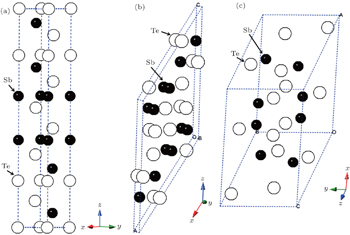Fig. 1. Unit cells for (a) phase I at 0 GPa, (b) phase II at 13.2 GPa, and (c) phase III at 20.5 GPa. Phase I is the hexagonal representation.Table 1.

The relaxed lattice constants of unit cell of phases I, II, and III under different pressures, together with some experimental results and calculated data. Note that our results of hexagon or unit cells are transformed from the calculated data of rhombohedron or primitive cells.

.

The values of lattice constant a obtained from our calculations accord well with the experimental values[14,16,19] with the errors less than 0.2% and the errors of lattice constant c are about −2.4%. For comparison between the theoretical and experimental results, we present the lattice parameters of phase II at 11.4 GPa and 13.2 GPa and phase III at 20.5 GPa. All these calculated parameters are relatively consistent with the former experimental and theoretical results, which indicate that the selected exchange–correlation functional and pseudopotential are reasonable as well.

To investigate the phase transition of Sb2Te3, Gibbs free energy GGibbs = Einternal+PVTS will be used, where Einternal, P, V, T, and S are internal energy, pressure, volume, temperature, and vibrational entropy, respectively. At a given pressure P and temperature T, the thermodynamic stable phase has the lowest Gibbs free energy in candidates. In our work, all the calculations are performed at zero K. Hence, the Gibbs free energy is equal to enthalpy H = Einternal+PV and the transition pressure is the value which the crosspoint between enthalpies of two competitive structures corresponds to. To find out transition pressures from phase I to phases II and III, values of enthalpy of three structures are calculated under the pressures in a range of 0 GPa–28 GPa in steps of 4 GPa. Pressure dependences of enthalpy are plotted in Fig. 2. As the pressure increases, the enthalpy of each structure increases linearly. Thus we perform the linear fitting for enthalpy of each structure and obtain linear equations as follows: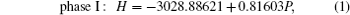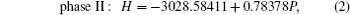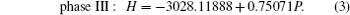Variations of enthalpy with pressure (a) for phase I to phase II, (b) for phase II to III.

Solving Eqs. (1) and (2), we obtain that the transition pressure from phase I to phase II is 9.4 GPa, which accords with experimental values 9.3 GPa, 9.8 GPa and 8 GPa. Similarly, according to Eqs. (2) and (3), we find that the change from phase II to phase III occurs at about 14.1 GPa, which is also consistent with experimental results 14.5 GPa, 15.2 GPa and 13.2 GPa. Moreover, the variations of volume per formula unit for the three phases are shown in Fig. 3. The volume of each phase decreases with increasing pressure. When the phase transition occurs, we can measure the relative volume collapse by using ΔVP/V0, where V0 is the ground state volume per formula unit. From phase I to phase II, ΔVP1/V0 is about 3.6%, and ΔVP2/V0 is about 2.6% from phase II to phase III.

 Figure Option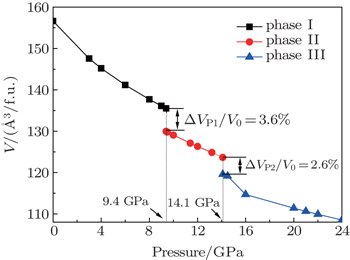Fig. 3. Variations of volume per formula unit with pressure for three phases.
3.2. Elastic properties

Elastic constant that relates stress to strain is of great importance for a material and helpful for understanding the acoustic velocity, bulk modulus and shear modulus. Therefore, we investigate the elastic constants of all three phases (0 GPa for phase I, 10 GPa for phase II and 15 GPa for phase III). As shown in Table 2, there are six independent elastic constants in the rhombohedral structure and the monoclinic phases have thirteen independent elastic constants.Table 2.

Calculated elastic constants Cij (GPa) of Sb2Te3 with phase I at 0 GPa, phase II at 10 GPa, and phase III at 15 GPa.

.

From the calculated elastic constants, we can obtain the Voigt average for bulk modulus (BV) and shear modulus (GV), together with the Reuss average for bulk modulus (BR) and shear modulus (GR).[28,29] According to the Voigt–Reuss–Hill approximations, the bulk modulus B and shear modulus G are defined as the arithmetic average of Reuss and Voight values: B = (BR + BV)/2, G = (GR + GV)/2. As shown in Table 3, at the ground state, the bulk modulus B of phase I is 42.1 GPa and in agreement with the experimental result 36.1 GPa and theoretical value 41.0 GPa. The bulk modulus B of phase II is 90.5 GPa at 10 GPa and 0 K and that of phase III is 121.3 GPa at 15 GPa and 0 K. From phase I to phase III, the value of B increases, implying that Sb2Te3 is more and more difficult to compress. Obviously, for each structure, its value of G (see Table 3) is smaller than B, which indicates that a shear deformation occurs more easily and shear modulus G is the parameter limiting the stability of Sb2Te3. The value of G of phase I is smaller than other phases, suggesting that the shear deformation is easier to occur in phase I. The shear modulus G is also an important parameter related to the hardness of a crystal. The value of G increases from phase I to phase III, indicating that the hardness of Sb2Te3 may increase under pressure. The brittleness or ductility has an important influence on applications of a material and there is an empirical criterion as follows: if B/G < 1.75, the material behaves as a brittle manner, otherwise, it behaves as a ductile manner. The values of B/G of phase I (1.67) and phase III (1.61) are less than the critical value 1.75, while the value of B/G for phase II (2.37) is larger than 1.75, which means that phases I and III are brittle, while phase II is ductile.Table 3.

Calculated values of bulk modulus B (GPa), shear modulus G (GPa) and B/G.

.

Young’s modulus E can measure stiffness of solid. The larger the value of E, the stiffer the material is. Poisson’s ratio σ gives information about the feature of bonding forces and Debye temperature Θ is a useful parameter and related to many physical properties, such as specific heat and melting temperature. Also it is usually believed that the quantum effect of material is remarkable below Debye temperature Θ, while it can be ignored above Debye temperature Θ. The Young’s modulus E, Poisson’s ratio σ, and Debye temperature Θ can be obtained from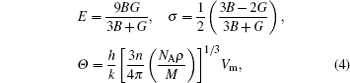where h is the Planck’s constant, k is the Boltzmann’s constant, NA is the Avogadro’s number, n is the number of atoms per formula unit, M is the molecular mass per formula unit, ρ is the density, and Vm is average acoustic velocity and can be calculated from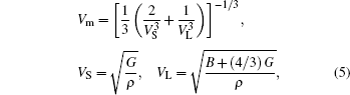where VS and VL are the shear and longitudinal acoustic velocity, respectively. The values of E, σ, Θ, VS, VL, and Vm are listed in Table 4. Obviously, the value of E increases gradually from phase I to phase III. This means that the stiffness is enhanced, as the pressure rises. If the value of σ is in a range of 0.25 −0.5, interatomic forces will be central. Thus phase I (σ = 0.2499) and phase III (σ = 0.2431) are noncentral force solids, while phase II (σ = 0.3148) is a central force solid. In addition, the values of VS are 1.95 km/s, 2.18 km/s, 2.93 km/s, respectively for phases I, II, and III. As for VL, they are 3.38 km/s, 4.19 km/s, 5.03 km/s, respectively. It is found that the values of VS and VL increase gradually from phase I to phase III, so the Vm increases (see Table 4). Using these data, we can obtain the Debye temperature Θ and it has the same increasing tendencies for phases I–III. At 80 K, the Debye temperature Θ is 160 K and a little smaller than our calculated result of 205 K. The values of Debye temperature Θ of phases II and III are 246 K and 337 K, respectively. However, there are no values of experimental Debye temperature Θ for phases II and III to be compared with each other.Table 4.

Values of longitudinal acoustic velocity VL (km/s), shear acoustic velocity VS (km/s), average acoustic velocity Vm (km/s), Debye temperature Θ (K), Young’s modulus E (GPa), Poisson’s ratio σ, and universal elastic anisotropy index Au of Sb2Te3.

.

Practically all elastic single crystals are anisotropic to a certain degree. Hence Au, a universal anisotropy index,[35,36] is employed to estimate the elastic anisotropy of Sb2Te3 and expressed as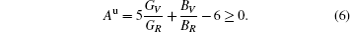The deviation of Au from zero indicates the extent of single crystal anisotropy and accounts for both the shear and the bulk contributions. As for Sb2Te3, the order of Au values is phase II (3.3) > phase I (2.9) > phase III (1.2), implying that of the three phases, phase II has the most serious degree of elastic anisotropy and phase III has the slightest one.

4. Electronic properties

The partial densities of states (PDOSs) of three phases, i.e., phase I at 0 GPa, phase II at 10 GPa and phase III at 15 GPa are shown in Fig. 4. For phase I (see Fig. 4(a)), the lower valence band (−14 eV–10.5 eV) is contributed from Sb-5s, Sb-5p, Te1-5s, and Te2-5s states. The middle valence band (−10 eV ∼ −7 eV) originates from Sb-5s, Te1-5s, Te2-5s, Te1-5p, and Te2-5p states in which the Sb-5s states are dominant. The upper valence band (−6 eV ∼ 0 eV) is mainly comprised of the contributions from Sb-5s, Sb-5p, Te1-5p, and Te2-5p states, and it is dominated by Sb-5p, Te1-5p, and Te2-5p states. In the conduction band, the Sb-5p, Te1-5p, and Te2-5p states play the dominant role in a range from 0 eV to 5 eV and the range from 5 eV to 9 eV consists of the constructions from Sb-5s, Te1-5s, and Te2-5s states. According to the analysis above, in the whole valence band, we discover that Sb-5s states hybridize with Te-5s states in an energy range from −14 eV to −7 eV and Sb-5p states strongly hybridize with Te-5p states from −6 eV to 0 eV. These hybridizations imply the existence of covalent bonding in α-Sb2Te3. For phase II (Fig. 4(b)), in the valence band, the energy range from −15 eV to −7 eV is mainly composed of the contributions from Sb1-5s, Sb2-5s, Te1-5s, Te2-5s, and Te3-5s states. The Sb1-5p, Sb2-5p, Te1-5p, Te2-5p, and Te3-5p states play a dominant role in an energy range from −7 eV to 5 eV. For phase III (Fig. 4(c)), Sb-5s, Te1-5s, and Te2-5s states contribute to the energy range from −15.5 eV to −7.5 eV. The energy range from −7 eV to 5 eV is dominated by Sb-5p, Te1-5p, and Te2-5p states. From the analysis, we can notice that the hybridization between Sb-5s and Te-5s states and hybridization between Sb-5p and Te-5p states also exist in phases II and phase III. These hybridizations also indicate that there exist the covalent bondings in β-Sb2Te3 and γ-Sb2Te3. It is found that energy range of conduction band of each of phases II and III is smaller than that of phase I, while energy ranges of valence band of phases II and III are slightly larger than that of phase I. Furthermore, the pressure-induced metallization is a well-known phenomenon in semiconductor. From the PDOSs of high pressure phases, we can infer that they exhibit a metallic behavior, which accords with the conclusion of other paper.

 Figure Option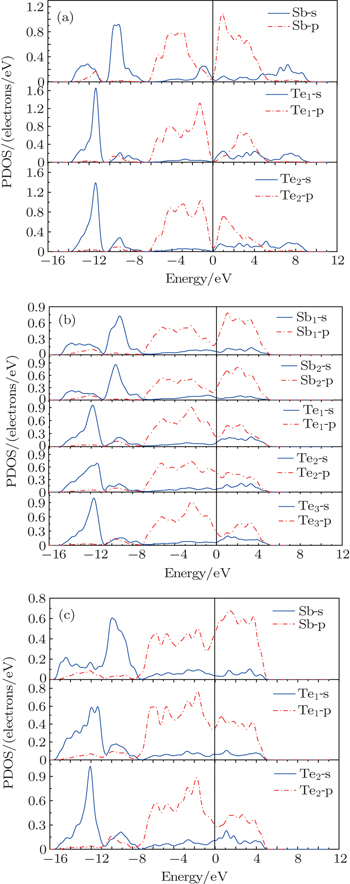Fig. 4. Calculated PDOS versus energy for (a) phase I at 0 GPa, (b) phase II at 10 GPa, and (c) phase III at 15 GPa.
5. Conclusions

In this work, we study the phase transitions, elastic and electronic properties of α-Sb2Te3 (R3m), β-Sb2Te3 (C2/m) and γ-Sb2Te3 (C2/c), by using first principle calculations based on the density functional theory. The calculated lattice constants of three phases are in agreement with their experimental values. The enthalpies of all the structures are calculated under a pressure range of 0 GPa–28 GPa. Solving the cross-point of enthalpy, we obtain the phase transition pressures to be 9.4 GPa for phases I to II and 14.1 GPa for phase II to phase III respectively. These results are consistent with experimental data. Elastic constants Cij, bulk modulus B, shear modulus G of the three phases are calculated and the obtained values of B/G indicate that phase I and III are brittle, while the phase II is ductile. Young’s modulus E, Poisson’s ratio σ, shear acoustic velocity VS, longitudinal acoustic velocity VL, average acoustic velocity Vm, and Debye temperature Θ are also studied and all these quantities increase from phase I to phase III, except the Poisson’s ratio σ. The universal anisotropy index Au suggests the order of degree of elastic anisotropy is phase II (3.3) > phase I (2.9) > phase III (1.2). In the end, the partial densities of states are studied, suggesting that there are some covalent features in the three phases and high pressure phases show metallic behaviors.

Reference
 1 Snyder G JTobere E S 2008 Nat. Mater. 7 105 2 Cao Y QZhao X BZhu T JZhang X BTu J P 2008 Appl. Phys. Lett. 92 143106 3 Kadel KKumari LLi W ZHuang J YProvencio P P 2011 Nanoscale Res. Lett. 6 57 4 Venkatasubramanian RSiivola EColpitts TO’Quinn B 2001 Nature 413 597 5 Thonhauser TScheidemantel T JSofo J OBadding J VMahan G D 2003 Phys. Rev. B 68 085201 6 Larson P 2006 Phys. Rev. B 74 205113 7 Yin YSone HHosaka S 2007 J. Appl. Phys. 102 064503 8 Zhang HLiu C XQi X LDai XFang ZZhang S C 2009 Nat. Phys. 5 438 9 Chen Y LAnalytis J GChu J HLiu Z KMo S KQi X LZhang H JLu D HDai XFang ZZhang S CFisher I RHussain ZShen Z X 2009 Science 325 178 10 Zhang WYu RZhang H JDai XFang Z 2010 New J. Phys. 12 065013 11 Zhang TCheng PChen XJia J FMa XHe KWang LZhang HDai XFang ZXie XXue Q K 2009 Phys. Rev. Lett. 103 266803 12 Einaga MTanabe YNakayama AOhmura AIshikawa FYamada Y 2010 J. Phys. Conf. Ser. 215 012036 13 Zhang J LZhang S JWeng H MZhang WYang L XLiu Q QFeng S MWang X CYu R CCao L ZWang LYang W GLiu H ZZhao W YZhang S CDai XFang ZJin C Q 2011 Proc. Natl. Acad. Sci. USA 108 24 14 Anderson T LKrause H B 1974 Acta Cryst. B 30 1307 15 Sakai NKajiwara TTakemura KMinomura SFujii Y 1981 Solid State Commun. 40 1045 16 Jacobsen M KKumar R SCornelius A LSinogeiken S VNicol M F 2007 AIP Conf. Proc. 955 171 17 Gomis OVilaplana RManjón F JRodríguez-Hernández PPérez-González EMu noz AKucek VDrasar C 2011 Phys. Rev. B 84 174305 18 Zhao JLiu HEhm LChen ZSinogeikin SZhao YGu G 2011 Inorg. Chem. 50 11291 19 Souza S MPoffo C MTrichês D MLima J CGrandi T APolian AGauthier M 2012 Physica B 407 3781 20 Ma YLiu GZhu PWang HWang XCui QLiu JMa Y 2012 J. Phys.: Condens. Matter 24 475403 21 Zhu LWang HWang YLv JMa YCui QMa YZou G 2011 Phys. Rev. Lett. 106 145501 22 Payne M CTeter M PAllen D CArias T AJoannopoulos J D 1992 Rev. Mod. Phys. 64 1045 23 Milman VWinkler BWhite J APackard C JPayne M CAkhmatskaya E VNobes R H 2000 Int. J. Quantum. Chem. 77 895 24 Perdew J PRuzsinszky ACsonka G IVydrov O AScuseria G EConstantin L AZhou XBurke K 2008 Phys. Rev. Lett. 100 136406 25 Perdew J PRuzsinszky ACsonka G IVydrov O AScuseria G E 2008 Phys. Rev. Lett. 101 239702 26 Vanderbilt D 1990 Phys. Rev. B 41 7892 27 Monkhorst H JPack J D 1976 Phys. Rev. B 13 5188 28 Berryman J GMech J 2005 Phys. Solids 53 2141 29 Wu ZZhao EXiang HHao XLiu XMeng J 2007 Phys. Rev. B 76 054115 30 Hill R 1952 Proc. Soc. London A 65 349 31 Pugh S F 1954 Philos. Mag. 45 823 32 Cheng CLv Z LCheng YJi G F 2014 J. Alloys Compd. 603 183 33 Chu FHe YThoma D JMitchell T E 1995 Scr. Metal. Mater. 33 1295 34 Dyck J SChen WUher CDrašar ČLošt’ák P 2002 Phys. Rev. B 66 125206 35 Ranganathan S IOstoja-Starzewski M 2008 Phys. Rev. Lett. 101 055504 36 Ding A LLi C MWang JAo JLi FChen Z Q 2014 Chin. Phys. B 23 096201